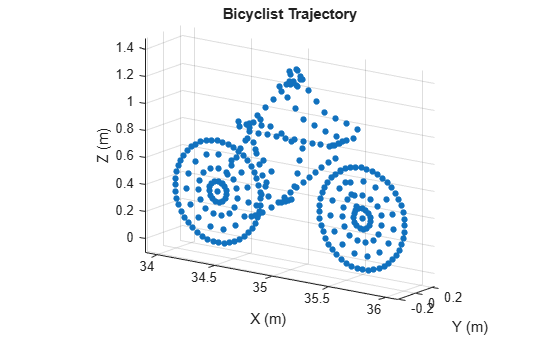# plot

Display locations of scatterers on bicyclist

Since R2019b

## Syntax

``plot(bicyclist)``
``fhndl = plot(bicyclist)``
``fhndl = plot(bicyclist,'Parent',ax)``

## Description

example

````plot(bicyclist)` displays the positions of all scatterers on a `bicyclist` at the current time. To display the current position of the bicyclist, call the `plot` object function after calling the `move` object function. Calling `plot` before any call to `move` displays the bicyclist at the origin.```
````fhndl = plot(bicyclist)` returns the figure handle of the display window.```
````fhndl = plot(bicyclist,'Parent',ax)` also specifies the plot axes for the bicyclist plot.```

## Examples

collapse all

Compute the backscattered radar signal from a bicyclist moving along the x-axis at 5 m/s away from a radar. Assume that the radar is located at the origin. The radar transmits an LFM signal at 24 GHz with a 300 MHz bandwidth. A signal is reflected at the moment the bicyclist starts to move and then one second later.

Initialize Bicyclist, Waveform, and Propagation Channel Objects

Initialize the `backscatterBicyclist`, `phased.LinearFMWaveform`, and `phased.FreeSpace` objects. Assume a 300 MHz sampling frequency. The initial position of the bicyclist lies on the x-axis 30 meters from the radar.

```bw = 300e6; fs = bw; fc = 24e9; radarpos = [0;0;0]; bpos = [30;0;0]; bicyclist = backscatterBicyclist( ... 'OperatingFrequency',fc,'NumWheelSpokes',15, ... 'InitialPosition',bpos,'Speed',5.0, ... 'InitialHeading',0.0); lfmwav = phased.LinearFMWaveform( ... 'SampleRate',fs, ... 'SweepBandwidth',bw); sig = lfmwav(); chan = phased.FreeSpace( ... 'OperatingFrequency',fc, ... 'SampleRate',fs, ... 'TwoWayPropagation',true);```

Plot Initial Bicyclist Position

Using the `move` object function, obtain the initial scatterer positions, velocities and the orientation of the bicyclist. Plot the initial position of the bicyclist. The `dt` argument of the `move` object function determines that the next call to `move` returns the bicyclist state of motion `dt` seconds later.

```dt = 1.0; [bpos,bvel,bax] = move(bicyclist,dt,0); plot(bicyclist)```Obtain First Reflected Signal

Propagate the signal to all scatterers and obtain the cumulative reflected return signal.

```N = getNumScatterers(bicyclist); sigtrns = chan(repmat(sig,1,N),radarpos,bpos,[0;0;0],bvel); [rngs,ang] = rangeangle(radarpos,bpos,bax); y0 = reflect(bicyclist,sigtrns,ang);```

Plot Bicyclist Position After Position Update

After the bicyclist has moved, obtain the scatterer positions and velocities and then move the bicycle along its trajectory for another second.

```[bpos,bvel,bax] = move(bicyclist,dt,0); plot(bicyclist)```Obtain Second Reflected Signal

Propagate the signal to all scatterers at their new positions and obtain the cumulative reflected return signal.

```sigtrns = chan(repmat(sig,1,N),radarpos,bpos,[0;0;0],bvel); [~,ang] = rangeangle(radarpos,bpos,bax); y1 = reflect(bicyclist,sigtrns,ang);```

Match Filter Reflected Signals

Match filter the reflected signals and plot them together.

```mfsig = getMatchedFilter(lfmwav); nsamp = length(mfsig); mf = phased.MatchedFilter('Coefficients',mfsig); ymf = mf([y0 y1]); fdelay = (nsamp-1)/fs; t = (0:size(ymf,1)-1)/fs - fdelay; c = physconst('LightSpeed'); plot(c*t/2,mag2db(abs(ymf))) ylim([-200 -50]) xlabel('Range (m)') ylabel('Magnitude (dB)') ax = axis; axis([0,100,ax(3),ax(4)]) grid legend('First pulse','Second pulse')```Compute the difference in range between the maxima of the two pulses.

```[maxy,idx] = max(abs(ymf)); dpeaks = t(1,idx(2)) - t(1,idx(1)); drng = c*dpeaks/2```
```drng = 4.9965 ```

The range difference is 5 m, as expected given the bicyclist speed.

## Input Arguments

collapse all

Bicyclist, specified as a `backscatterBicyclist` object.

Plot axes, specified as an axes handle.

Data Types: `double`

## Output Arguments

collapse all

Figure handle of plot window.

## Version History

Introduced in R2019b